# Summary

This R Markdown document provides many different examples for creating plots with rpact and ggplot2, e.g. the `plot` arguments `type` and `showSource` will be illustrated.

# 1 Design plots

``````library(rpact)
packageVersion("rpact") # version should be version 2.0.5 or later``````
``##  '2.0.6'``

## 1.1 One-sided design with futility bounds

``````design <- getDesignGroupSequential(kMax = 3,
typeOfDesign = "OF", sided = 1,
futilityBounds = c(0, 0.1))

plot(design, type = 1, showSource = TRUE)``````
``````## Source data of the plot:
##   x-axis: design\$informationRates
##   y-axes:
##     y1: design\$futilityBounds
##     y2: design\$criticalValues``````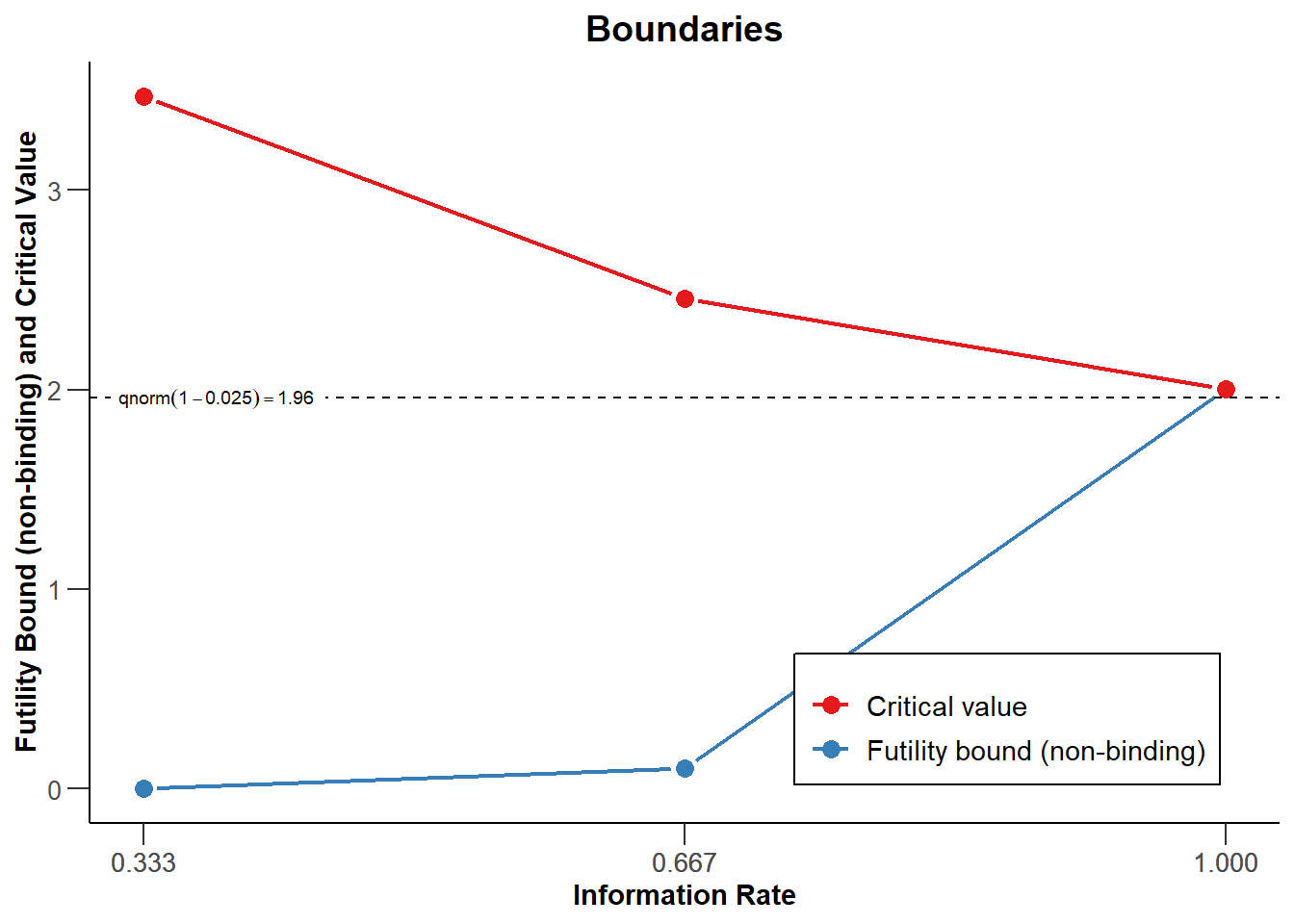``plot(design, type = 3, showSource = TRUE)``
``````## Source data of the plot:
##   x-axis: design\$informationRates
##   y-axis: design\$stageLevels``````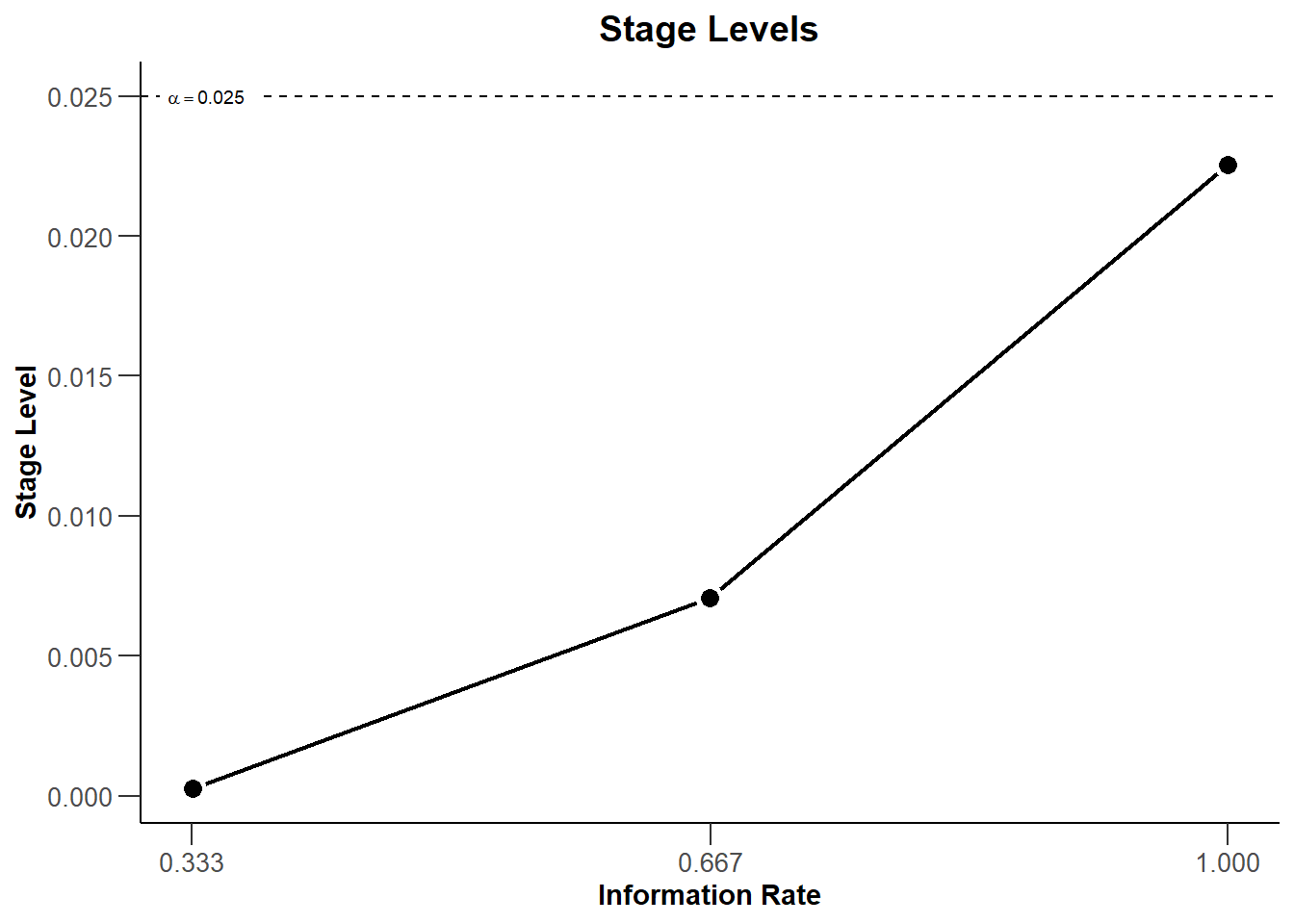``plot(design, type = 4, showSource = TRUE)``
``````## Source data of the plot:
##   x-axis: design\$informationRates
##   y-axis: design\$alphaSpent``````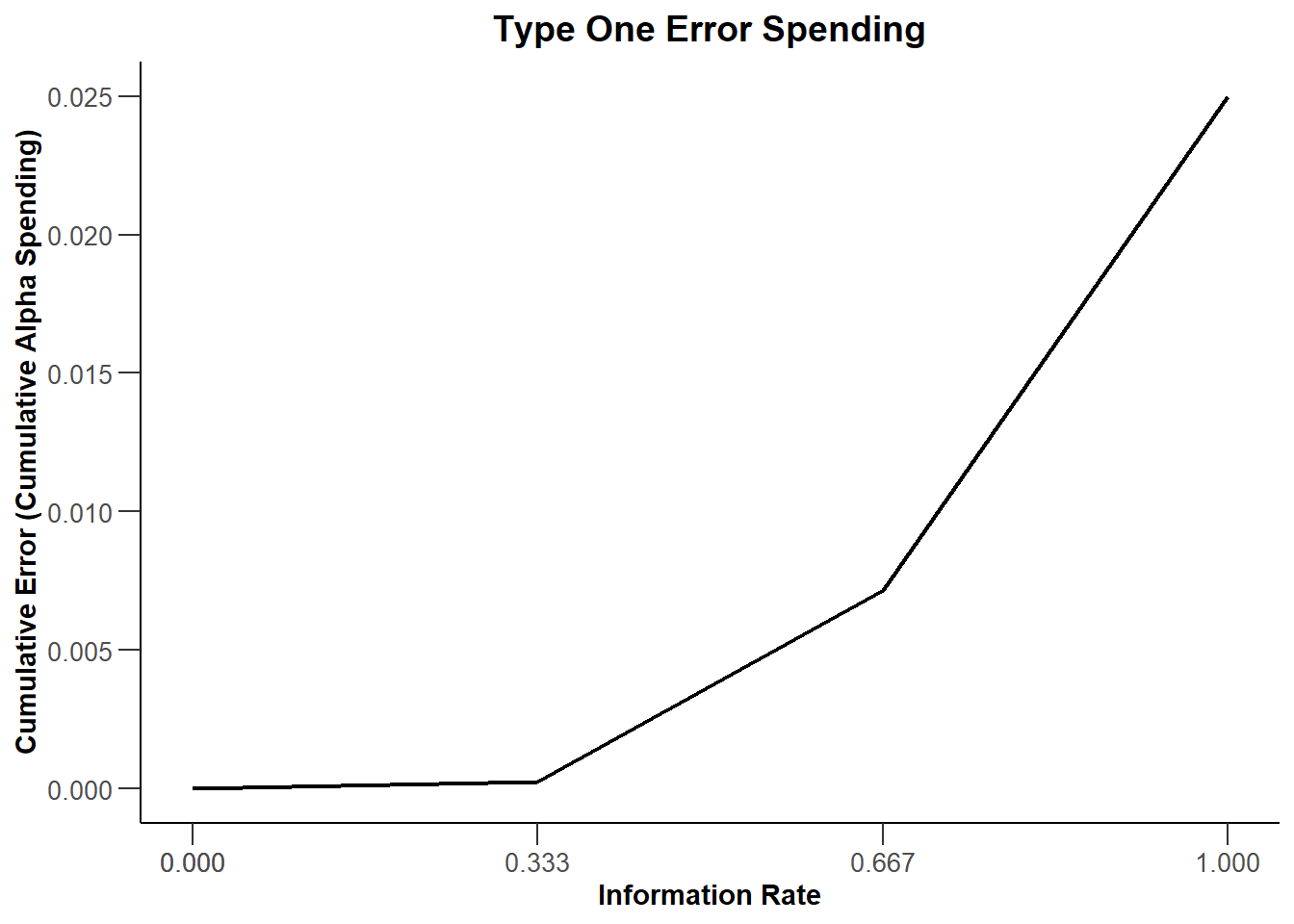``plot(design, type = 5, nMax = 10, showSource = TRUE) ``
``````## Source data of the plot:
##   x-axis: design\$theta
##   y-axes:
##     y1: design\$overallEarlyStop
##     y2: design\$calculatedPower``````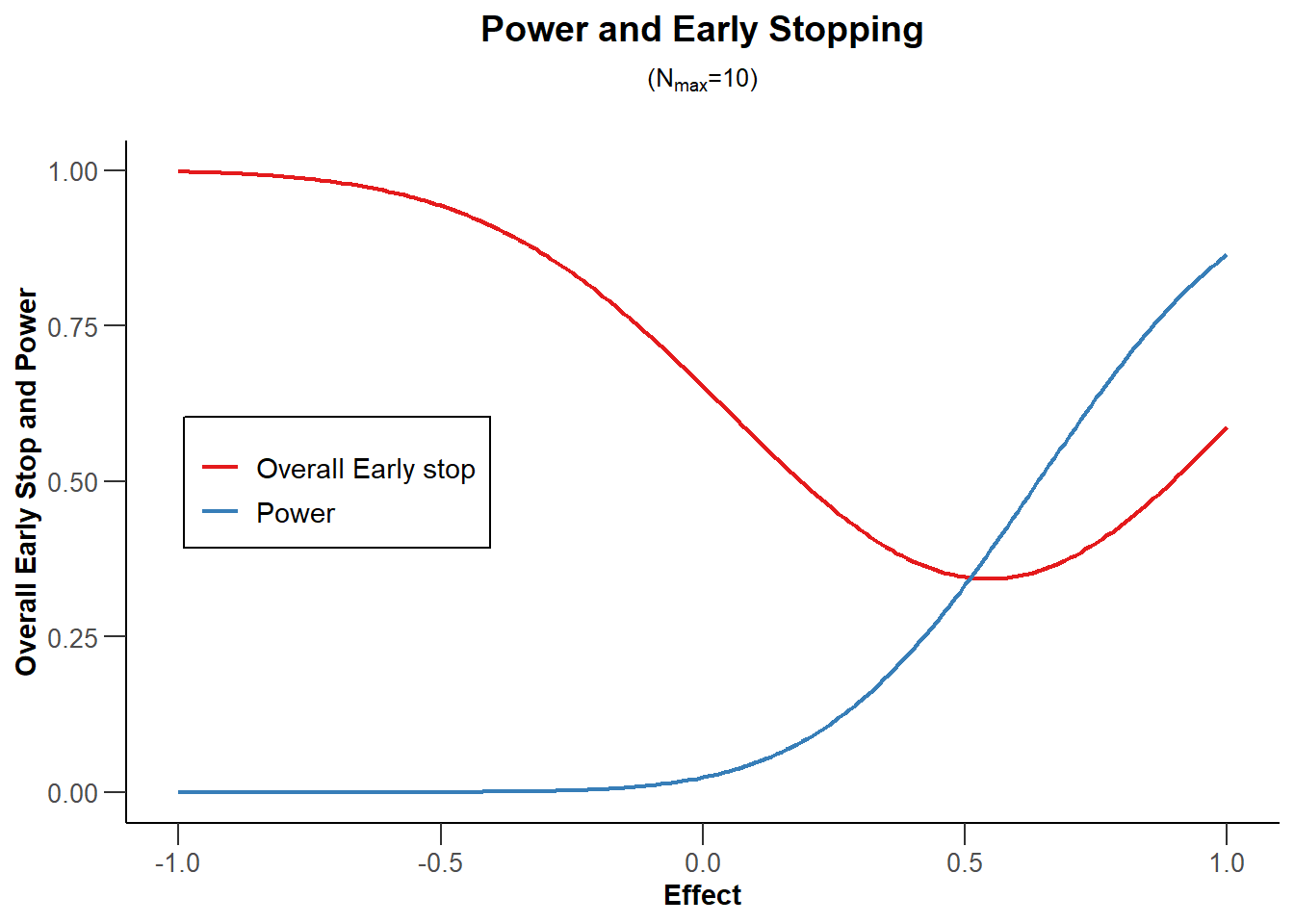``plot(design, type = 6, nMax = 10, showSource = TRUE)``
``````## Source data of the plot:
##   x-axis: design\$theta
##   y-axes:
##     y1: design\$averageSampleNumber
##     y2: design\$overallEarlyStop
##     y3: design\$calculatedPower``````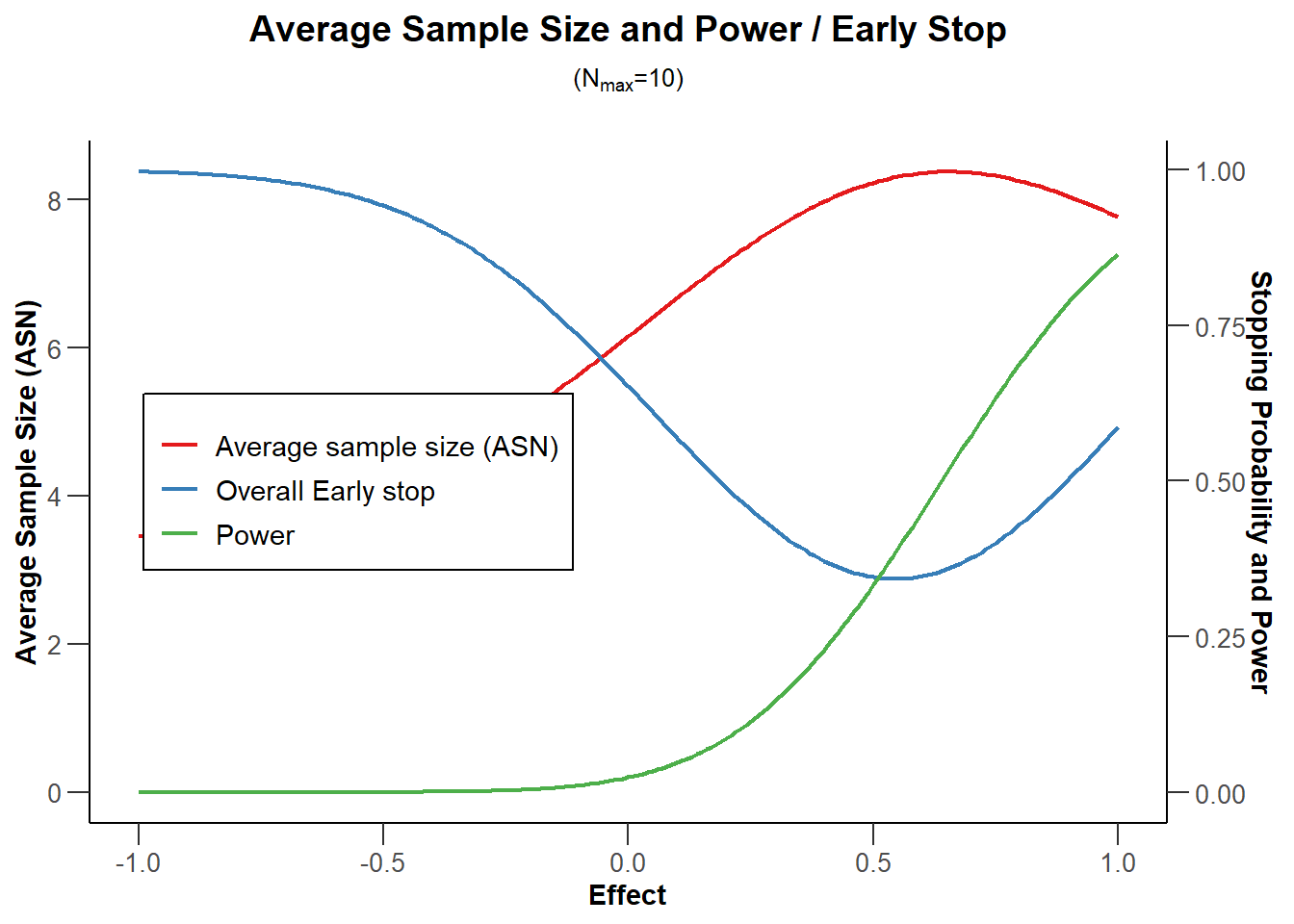``plot(design, type = 7, nMax = 10, showSource = TRUE)``
``````## Source data of the plot:
##   x-axis: design\$theta
##   y-axis: design\$calculatedPower``````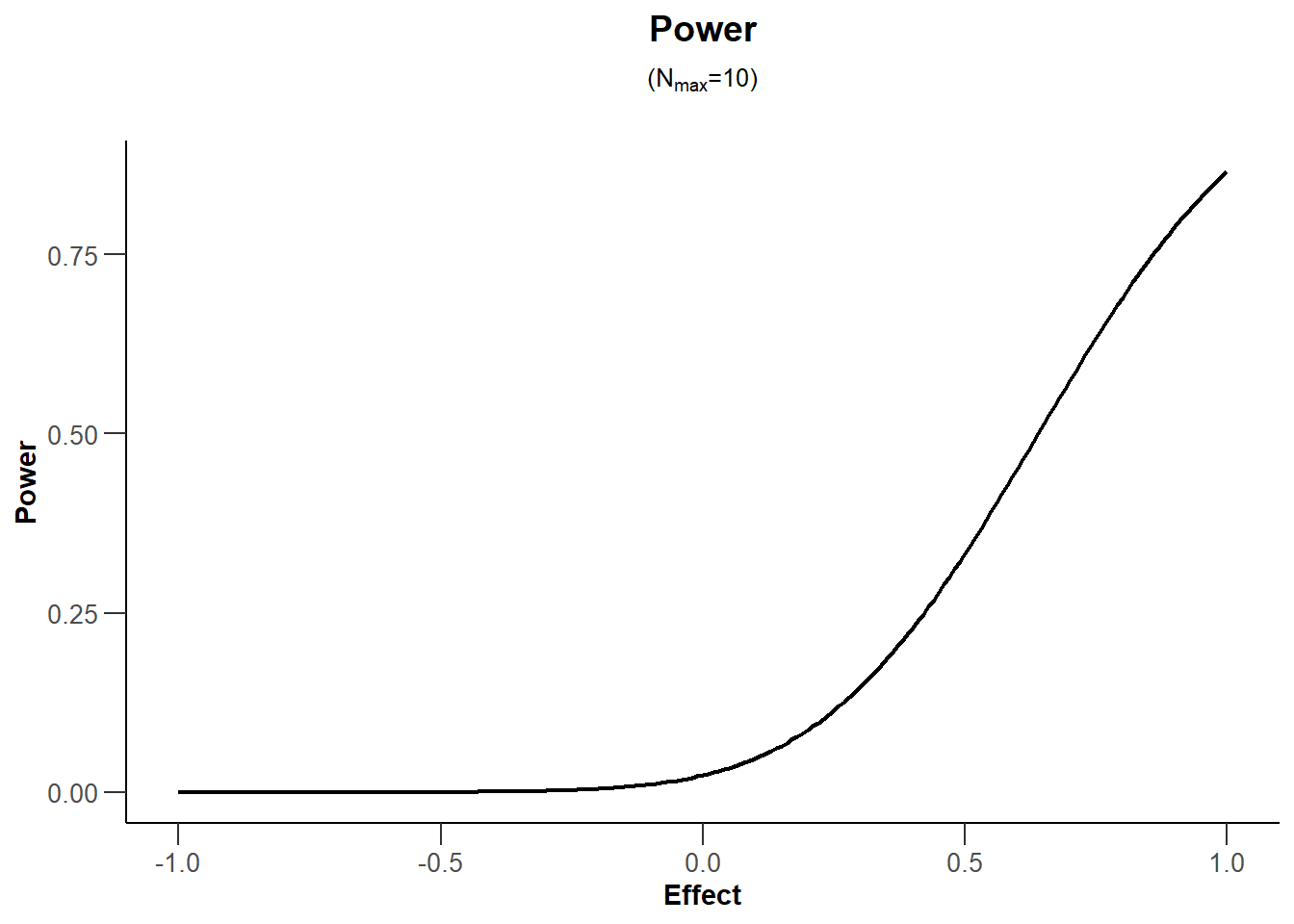``plot(design, type = 8, nMax = 10, showSource = TRUE)``
``````## Source data of the plot:
##   x-axis: design\$theta
##   y-axis: design\$overallEarlyStop``````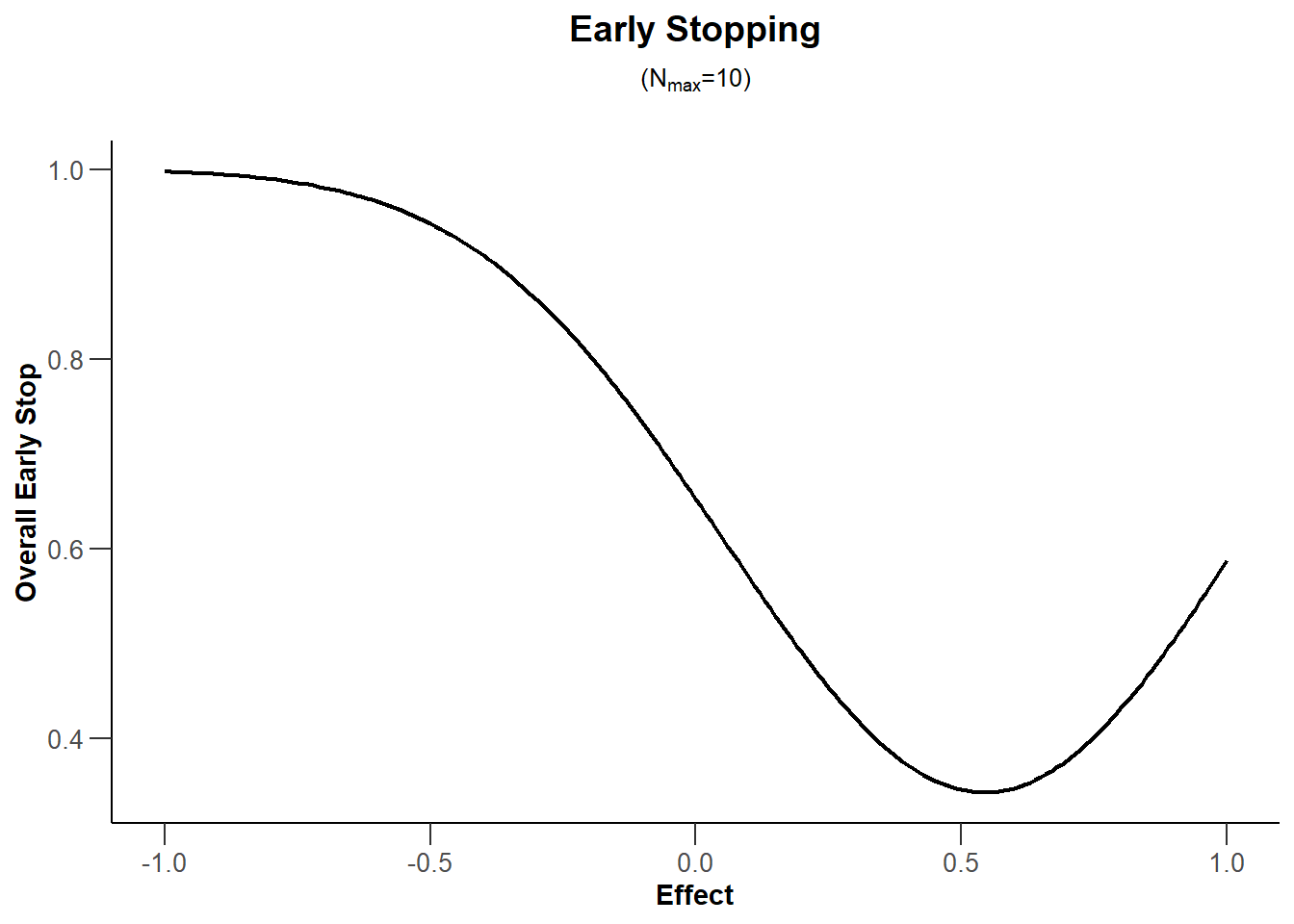``plot(design, type = 9, nMax = 10, showSource = TRUE)``
``````## Source data of the plot:
##   x-axis: design\$theta
##   y-axis: design\$averageSampleNumber``````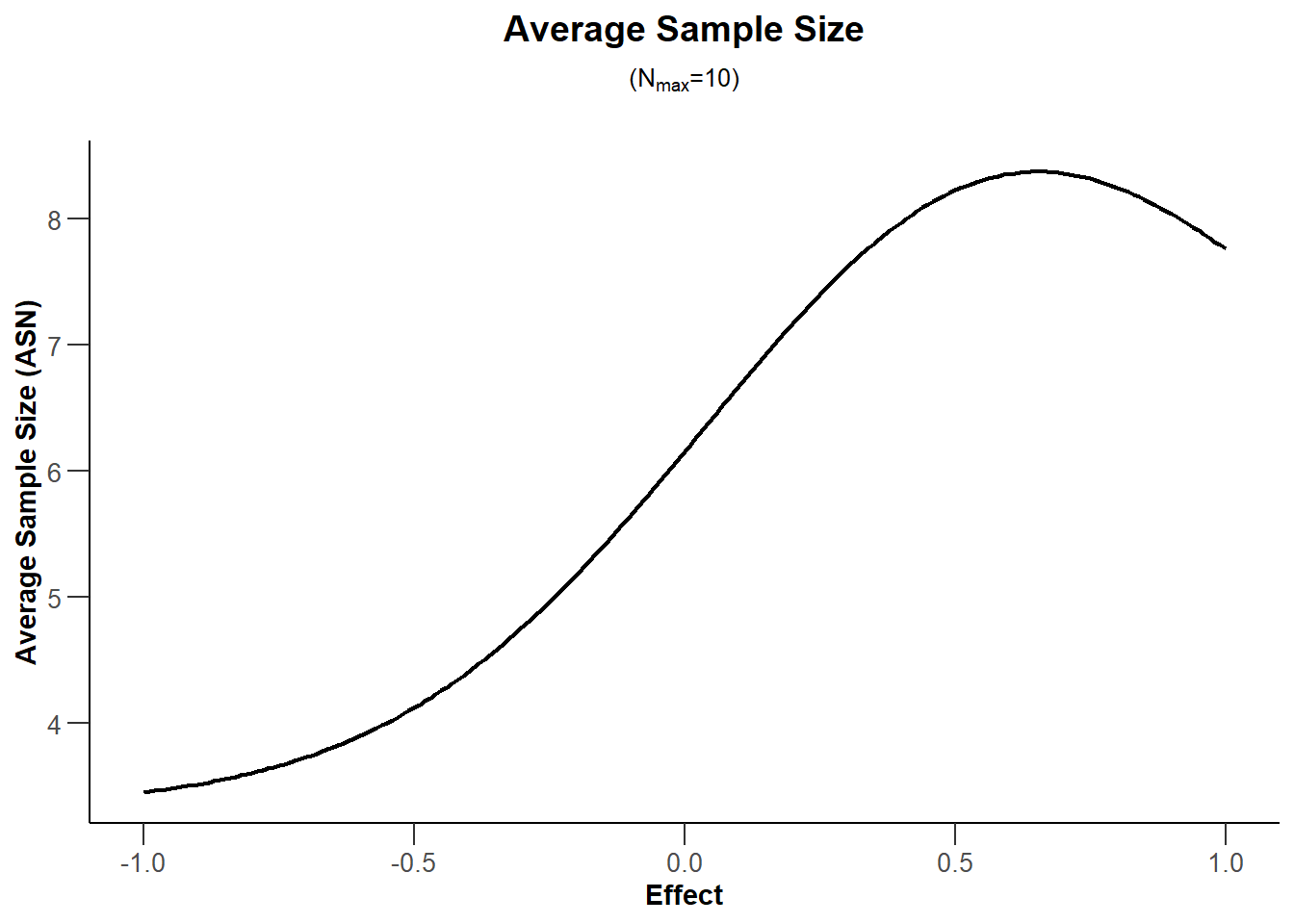## 1.2 Two-sided design

``````design <- getDesignGroupSequential(
kMax = 4, typeOfDesign = "OF", sided = 2, twoSidedPower = TRUE)

plot(design, type = 1)``````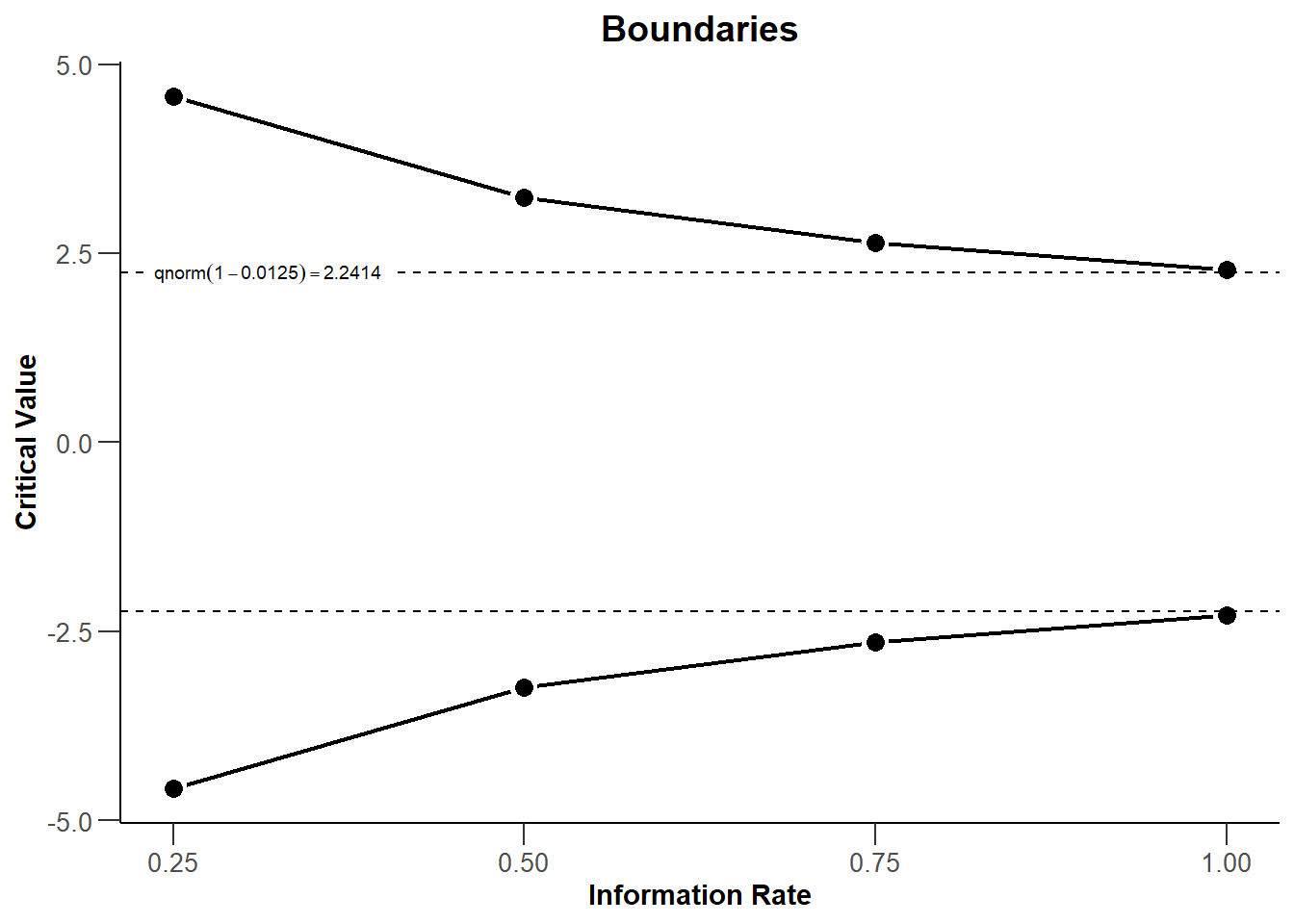``plot(design, type = 3)``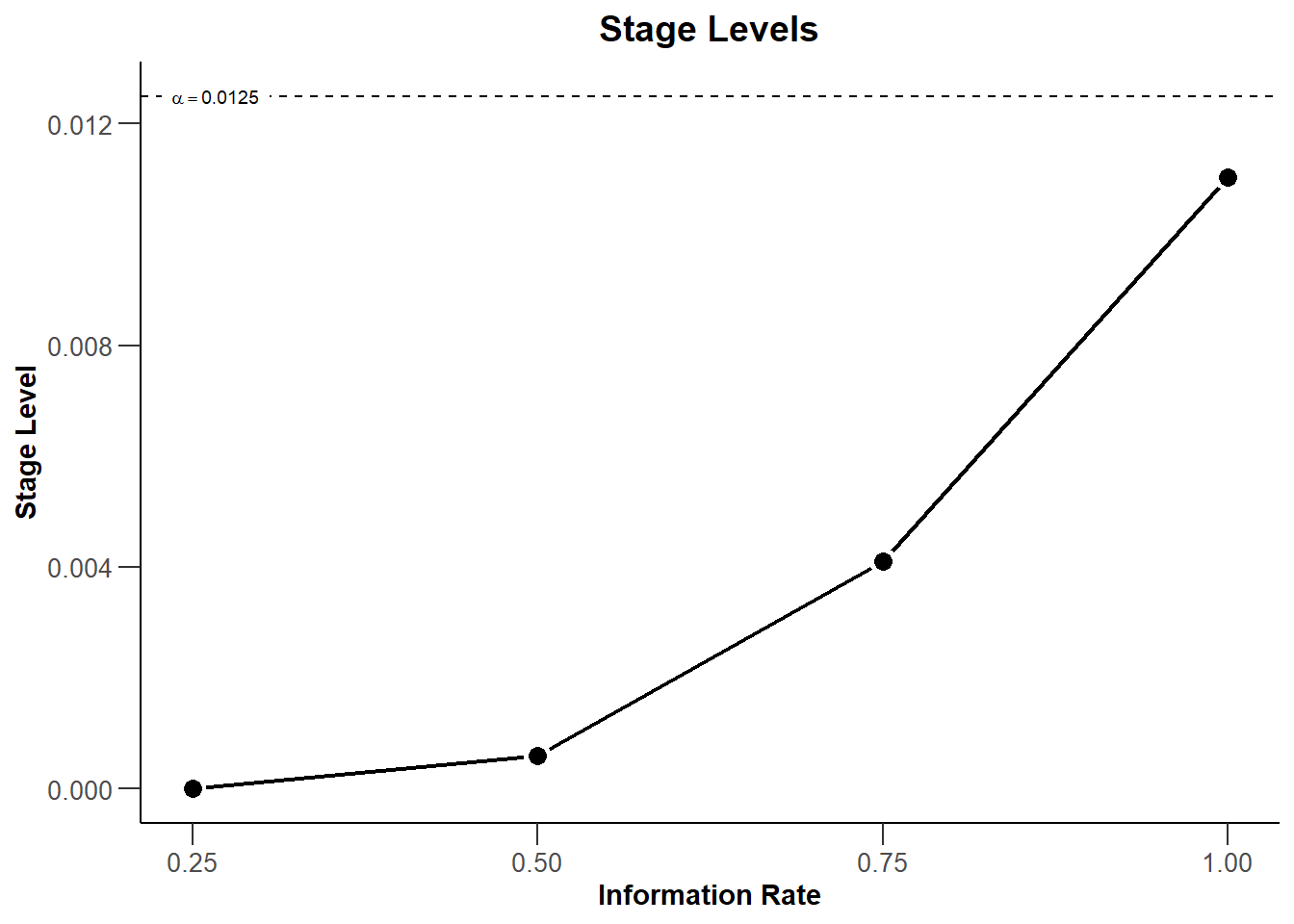``plot(design, type = 4)``